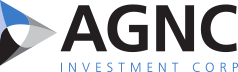Print Page     Close Window

## SEC Filings

 10-Q AGNC INVESTMENT CORP. filed this Form 10-Q on 08/05/2016 Entire Document

_______________________
* Except as noted below, average numbers for each period are weighted based on days on our books and records. All percentages are annualized.

 1. Net asset value per common share is calculated as our total stockholders' equity, less our Series A and Series B Preferred Stock aggregate liquidation preference, divided by our number of common shares outstanding as of period end.
 2. We voluntarily discontinued hedge accounting for our interest rate swaps as of September 30, 2011. Please refer to our Interest Expense and Cost of Funds discussion in Management's Discussion and Analysis of Financial Condition and Results of Operations and Notes 3 and 6 of our Consolidated Financial Statements in this Quarterly Report on Form 10-Q for additional information regarding our discontinuance of hedge accounting.
 3. Average mortgage borrowings include agency repo, FHLB advances and debt of consolidated VIEs. Amount excludes U.S. Treasury repo agreements and TBA contracts.
 4. Average stockholders' equity calculated as our average month-end stockholders' equity during the period.
 5. Average coupon for the period was calculated by dividing our total coupon (or cash) interest income on investment securities by our average investment securities held at par.
 6. Average asset yield for the period was calculated by dividing our total cash interest income on investment securities, adjusted for amortization of premiums and discounts, by our average amortized cost of investment securities held.
 7. Average cost of funds includes mortgage borrowings and interest rate swap periodic costs. Amount excludes interest rate swap termination fees, forward starting swaps and costs associated with other supplemental hedges, such as interest rate swaptions and U.S. Treasury positions. Average cost of funds for the period was calculated by dividing our total cost of funds by our average mortgage borrowings outstanding for the period.
 8. TBA dollar roll income / (loss) is net of short TBAs used for hedging purposes. Dollar roll income excludes the impact of other supplemental hedges, and is recognized in gain (loss) on derivative instruments and other securities, net.
 9. Average cost of funds as of period end includes mortgage borrowings outstanding, plus the impact of interest rate swaps. Amount excludes costs associated with other supplemental hedges such as swaptions, U.S. Treasuries and TBA positions.
 10. Net comprehensive income (loss) return on average common equity for the period was calculated by dividing our comprehensive income/(loss) available /(attributable) to common stockholders by our average stockholders' equity, net of the Series A and Series B Preferred Stock aggregate liquidation preference.
 11. Economic return (loss) on common equity represents the sum of the change in our net asset value per common share and our dividends declared on common stock during the period over our beginning net asset value per common share.
 12. Average leverage during the period was calculated by dividing our daily weighted average mortgage borrowings outstanding for the period by the sum of our average stockholders' equity less our average investment in REIT equity securities for the period. Leverage excludes U.S. Treasury repurchase agreements.
 13. Average "at risk" leverage, inclusive of net TBA portfolio, during the period includes the components of "average leverage" plus our daily weighted average net TBA dollar position (at cost) during the period.
 14. Leverage at period end is calculated by dividing the sum of our mortgage borrowings outstanding and our receivable / payable for unsettled investment securities by the sum of our total stockholders' equity less the fair value of investments in REIT equity securities at period end. Leverage excludes U.S. Treasury repurchase agreements.
 15. "At risk" leverage at period end, inclusive of net TBA position, includes the components of "leverage (as of period end)" plus our net TBA dollar roll position outstanding as of period end, at cost.

Interest Income and Asset Yield
The following table summarizes our interest income for the three and six months ended June 30, 2016 and 2015 (dollars in millions):
 Three Months Ended June 30, Six Months Ended June 30, 2016 2015 2016 2015 Amount Yield Amount Yield Amount Yield Amount Yield Cash/coupon interest income \$ 452 3.63 % \$ 483 3.60 % \$ 897 3.63 % \$ 999 3.62 % Net premium amortization (134 ) (1.17 )% (69 ) (0.65 )% (284 ) (1.24 )% (202 ) (0.86 )% Interest income \$ 318 2.46 % \$ 414 2.95 % \$ 613 2.39 % \$ 797 2.76 % Weighted average actual portfolio CPR for securities held during the period 12 % 12 % 10 % 10 % Weighted average projected CPR for the remaining life of securities held as of period end 11 % 8 % 11 % 8 % Average 30-year fixed rate mortgage rate as of period end 1 3.48 % 4.08 % 3.48 % 4.08 % 10-year U.S. Treasury rate as of period end 1.49 % 2.33 % 1.49 % 2.33 %
_______________________
 1. Source: Freddie Mac Primary Fixed Mortgage Rate Mortgage Market Survey

The principal elements impacting our interest income are the size of our average investment portfolio and the yield on our investments. The following is a summary of the estimated impact of each of these elements on the decline in interest income compared to the prior year period for the three and six months ended June 30, 2016 (in millions):

38